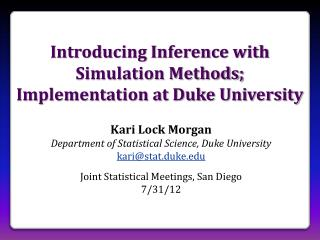# Introducing Inference with Simulation Methods; Implementation at Duke University - PowerPoint PPT PresentationDownload PresentationIntroducing Inference with Simulation Methods; Implementation at Duke University

Introducing Inference with Simulation Methods; Implementation at Duke University
Download Presentation## Introducing Inference with Simulation Methods; Implementation at Duke University

- - - - - - - - - - - - - - - - - - - - - - - - - - - E N D - - - - - - - - - - - - - - - - - - - - - - - - - - -
##### Presentation Transcript

1. Introducing Inference with Simulation Methods; Implementation at Duke University Kari Lock Morgan Department of Statistical Science, Duke University kari@stat.duke.edu Joint Statistical Meetings, San Diego 7/31/12

2. Methods of Inference • Simulation Methods (bootstrap, randomization) • intrinsically connected to the concepts • minimal background knowledge needed • same procedure applies to all statistics • no conditions to check • Traditional Methods (normal, t-distribution) • familiarity expected after intro stat • Use simulation methods to introduce inference, and then teach the traditional methods as “short-cut formulas”

3. Topics • Introduction to Data • Collecting data • Describing data • Introduction to Inference • Confidence intervals (bootstrap) • Hypothesis tests (randomization) • Normal and t-based methods • Chi-square and ANOVA • Randomization and theoretical approaches • Regression

4. Sleep versus Caffeine • Students were given words to memorize, then randomly assigned to take either a 90 min nap, or a caffeine pill. 2 ½ hours later, they were tested on their recall ability. • words • Is sleep better than caffeine for memory? Mednick, Cai, Kanady, and Drummond (2008). “Comparing the benefits of caffeine, naps and placebo on verbal, motor and perceptual memory,” Behavioral Brain Research, 193, 79-86.

5. Traditional Inference Conditions Met? 2. Which formula? 5. Which theoretical distribution? 6. df? 7. find p-value 3. Calculate numbers and plug into formula 0.025 < p-value < 0.05 > pt(2.14, 11, lower.tail=FALSE)  0.02780265 4. Plug into calculator

6. Simulation Inference • How extreme would a sample difference of 3 be, if there were no difference between sleep and caffeine for word recall? • What kind of differences would we observe, just by random chance? • Simulate many randomizations, assuming no difference • Calculate the p-value as the proportion of simulated randomizations that yield differences as extreme as the observed 3

7. Randomization Test StatKey at www.lock5stat.com Distribution of Statistic Assuming Null is True Proportion as extreme as observed statistic p-value observed statistic

8. Theoretical Approach • Normal and t-based inference afterbootstrapping and randomization: • Students have seen the normal distribution repeatedly – CLT easy! • Same idea, just using formula for SE and comparing to theoretical distribution • Can go very quickly through this!

9. Theoretical Approach p-value t-statistic

10. Chi-Square and ANOVA • Introduce new statistic - 2 or F • Students know that these can be compared to either a randomization distribution or a theoretical distribution • Students are comfortable using either method, and see the connection! • If conditions are met, the randomization and theoretical distributions are the same!

11. Chi-Square Statistic Randomization Distribution p-value = 0.357 Chi-Square Distribution (3 df) p-value = 0.356 2statistic = 3.242 2statistic = 3.242

12. Student Preferences • Which way did you prefer to learn inference (confidence intervals and hypothesis tests)?

13. Student Behavior • Students were given data on the second midterm and asked to compute a confidence interval for the mean • How they created the interval:

14. A Student Comment " I took AP Stat in high school and I got a 5.  It was mainly all equations, and I had no idea of the theory behind any of what I was doing.Statkey and bootstrapping really made me understand the concepts I was learning, as opposed to just being able to just spit them out on an exam.” - one of my students

15. Further Information • Want more information on teaching with this approach? • www.lock5stat.com • Questions? • kari@stat.duke.edu